Next: Axially Symmetric Mass Distributions Up: Gravitational Potential Theory Previous: Introduction

Gravitational Potential

Consider two point masses,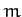and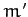, located at position vectors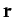and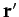, respectively. According to Section 5.3, the acceleration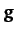of massdue to the gravitational force exerted on it by masstakes the form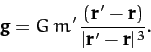(861)

Now, the-component of this acceleration is written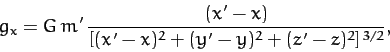(862)

where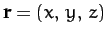and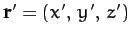. However, as is easily demonstrated,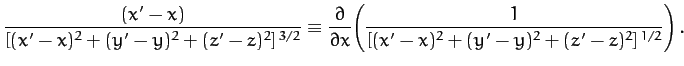(863)

Hence,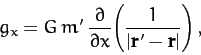(864)

with analogous expressions for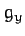and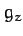. It follows that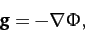(865)

where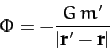(866)

is termed the gravitational potential. Of course, we can only writein the form (865) because gravity is a conservative force--see Chapter 2. Note that gravitational potential,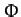, is directly related to gravitational potential energy,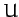. In fact, the potential energy of massis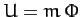.

Now, it is well-known that gravity is a superposable force. In other words, the gravitational force exerted on some test mass by a collection of point masses is simply the sum of the forces exerted on the test mass by each point mass taken in isolation. It follows that the gravitational potential generated by a collection of point masses at a certain location in space is the sum of the potentials generated at that location by each point mass taken in isolation. Hence, using Equation (866), if there are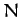point masses,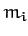(for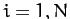), located at position vectors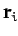, then the gravitational potential generated at position vectoris simply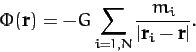(867)

Suppose, finally, that, instead of having a collection of point masses, we have a continuous mass distribution. In other words, let the mass at position vectorbe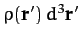, where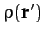is the local mass density, and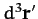a volume element. Summing over all space, and taking the limit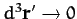, Equation (867) yields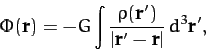(868)

where the integral is taken over all space. This is the general expression for the gravitational potential,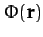, generated by a continuous mass distribution,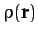.Next: Axially Symmetric Mass Distributions Up: Gravitational Potential Theory Previous: Introduction
Richard Fitzpatrick 2011-03-31